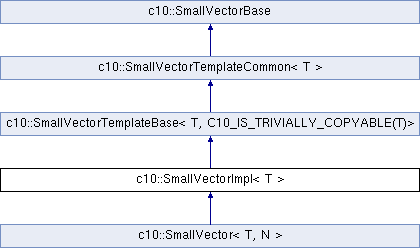Caffe2 - C++ API A deep learning, cross platform ML framework
c10::SmallVectorImpl< T > Class Template Reference

This class consists of common code factored out of the SmallVector class to reduce code duplication based on the SmallVector 'N' template parameter. More...

`#include <SmallVector.h>`

Inheritance diagram for c10::SmallVectorImpl< T >:## Public Types

using iterator = typename SuperClass::iterator

using const_iterator = typename SuperClass::const_iterator

using size_type = typename SuperClass::size_typePublic Types inherited from c10::SmallVectorTemplateCommon< T >
using size_type = size_t

using difference_type = ptrdiff_t

using value_type = T

using iterator = T *

using const_iterator = const T *

using const_reverse_iterator = std::reverse_iterator< const_iterator >

using reverse_iterator = std::reverse_iterator< iterator >

using reference = T &

using const_reference = const T &

using pointer = T *

using const_pointer = const T *

## Public Member Functions

SmallVectorImpl (const SmallVectorImpl &)=delete

void clear ()

void resize (size_type N)

void resize (size_type N, const T &NV)

void reserve (size_type N)

T pop_back_val ()

void swap (SmallVectorImpl &RHS)

template<typename in_iter , typename = typename std::enable_if<std::is_convertible< typename std::iterator_traits<in_iter>::iterator_category, std::input_iterator_tag>::value>::type>
void append (in_iter in_start, in_iter in_end)
Add the specified range to the end of the SmallVector.

void append (size_type NumInputs, const T &Elt)
Add the specified range to the end of the SmallVector.

void append (std::initializer_list< T > IL)

void assign (size_type NumElts, const T &Elt)

template<typename in_iter , typename = typename std::enable_if<std::is_convertible< typename std::iterator_traits<in_iter>::iterator_category, std::input_iterator_tag>::value>::type>
void assign (in_iter in_start, in_iter in_end)

void assign (std::initializer_list< T > IL)

iterator erase (const_iterator CI)

iterator erase (const_iterator CS, const_iterator CE)

iterator insert (iterator I, T &&Elt)

iterator insert (iterator I, const T &Elt)

iterator insert (iterator I, size_type NumToInsert, const T &Elt)

template<typename ItTy , typename = typename std::enable_if<std::is_convertible< typename std::iterator_traits<ItTy>::iterator_category, std::input_iterator_tag>::value>::type>
iterator insert (iterator I, ItTy From, ItTy To)

void insert (iterator I, std::initializer_list< T > IL)

template<typename... ArgTypes>
void emplace_back (ArgTypes &&...Args)

SmallVectorImploperator= (const SmallVectorImpl &RHS)

SmallVectorImploperator= (SmallVectorImpl &&RHS)

bool operator== (const SmallVectorImpl &RHS) const

bool operator!= (const SmallVectorImpl &RHS) const

bool operator< (const SmallVectorImpl &RHS) const

void set_size (size_type N)
Set the array size to `N`, which the current array must have enough capacity for. More...Public Member Functions inherited from c10::SmallVectorTemplateBase< T, C10_IS_TRIVIALLY_COPYABLE(T)>
void push_back (const T &Elt)

void push_back (T &&Elt)

void pop_back ()Public Member Functions inherited from c10::SmallVectorTemplateCommon< T >
iterator begin ()

const_iterator begin () const

iterator end ()

const_iterator end () const

reverse_iterator rbegin ()

const_reverse_iterator rbegin () const

reverse_iterator rend ()

const_reverse_iterator rend () const

size_type size () const

size_type max_size () const

size_t capacity () const
Return the total number of elements in the currently allocated buffer.

pointer data ()
Return a pointer to the vector's buffer, even if empty().

const_pointer data () const
Return a pointer to the vector's buffer, even if empty().

reference at (size_type idx)

const_reference at (size_type idx) const

reference operator[] (size_type idx)

const_reference operator[] (size_type idx) const

reference front ()

const_reference front () const

reference back ()

const_reference back () constPublic Member Functions inherited from c10::SmallVectorBase
size_t size_in_bytes () const
This returns size()*sizeof(T).

size_t capacity_in_bytes () const
capacity_in_bytes - This returns capacity()*sizeof(T).

bool empty () const

## Protected Member Functions

SmallVectorImpl (unsigned N)Protected Member Functions inherited from c10::SmallVectorTemplateBase< T, C10_IS_TRIVIALLY_COPYABLE(T)>
SmallVectorTemplateBase (size_t Size)

void grow (size_t MinSize=0)
Grow the allocated memory (without initializing new elements), doubling the size of the allocated memory. More...Protected Member Functions inherited from c10::SmallVectorTemplateCommon< T >
SmallVectorTemplateCommon (size_t Size)

void grow_pod (size_t MinSizeInBytes, size_t TSize)

bool isSmall () const
Return true if this is a smallvector which has not had dynamic memory allocated for it. More...

void resetToSmall ()
Put this vector in a state of being small.

void setEnd (T *P)

iterator capacity_ptr ()

const_iterator capacity_ptr () constProtected Member Functions inherited from c10::SmallVectorBase
SmallVectorBase (void *FirstEl, size_t Size)

void grow_pod (void *FirstEl, size_t MinSizeInBytes, size_t TSize)
This is an implementation of the grow() method which only works on POD-like data types and is out of line to reduce code duplication. More...Static Protected Member Functions inherited from c10::SmallVectorTemplateBase< T, C10_IS_TRIVIALLY_COPYABLE(T)>
static void destroy_range (T *S, T *E)

static void uninitialized_move (It1 I, It1 E, It2 Dest)
Move the range [I, E) into the uninitialized memory starting with "Dest", constructing elements as needed. More...

static void uninitialized_copy (It1 I, It1 E, It2 Dest)
Copy the range [I, E) onto the uninitialized memory starting with "Dest", constructing elements as needed. More...Protected Attributes inherited from c10::SmallVectorBase
void * BeginX

void * EndX

void * CapacityX

## Detailed Description

### template<typename T> class c10::SmallVectorImpl< T >

This class consists of common code factored out of the SmallVector class to reduce code duplication based on the SmallVector 'N' template parameter.

Definition at line 382 of file SmallVector.h.

## Member Function Documentation

template<typename T>
 void c10::SmallVectorImpl< T >::set_size ( size_type N )
inline

Set the array size to `N`, which the current array must have enough capacity for.

This does not construct or destroy any elements in the vector.

Clients can use this in conjunction with capacity() to write past the end of the buffer when they know that more elements are available, and only update the size later. This avoids the cost of value initializing elements which will only be overwritten.

Definition at line 757 of file SmallVector.h.

The documentation for this class was generated from the following file: